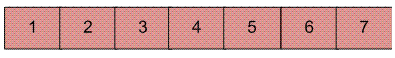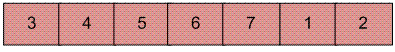Open in App
Not now

# Javascript Program for Reversal algorithm for array rotation

• Last Updated : 28 May, 2022

Write a function rotate(arr[], d, n) that rotates arr[] of size n by d elements.
Example :

```Input :  arr[] = [1, 2, 3, 4, 5, 6, 7]
d = 2
Output : arr[] = [3, 4, 5, 6, 7, 1, 2] ```Rotation of the above array by 2 will make arrayThe first 3 methods to rotate an array by d elements has been discussed in this post.
Method 4 (The Reversal Algorithm) :
Algorithm :

```rotate(arr[], d, n)
reverse(arr[], 1, d) ;
reverse(arr[], d + 1, n);
reverse(arr[], 1, n);```

Let AB are the two parts of the input array where A = arr[0..d-1] and B = arr[d..n-1]. The idea of the algorithm is :

• Reverse A to get ArB, where Ar is reverse of A.
• Reverse B to get ArBr, where Br is reverse of B.
• Reverse all to get (ArBr) r = BA.

Example :
Let the array be arr[] = [1, 2, 3, 4, 5, 6, 7], d =2 and n = 7
A = [1, 2] and B = [3, 4, 5, 6, 7]

• Reverse A, we get ArB = [2, 1, 3, 4, 5, 6, 7]
• Reverse B, we get ArBr = [2, 1, 7, 6, 5, 4, 3]
• Reverse all, we get (ArBr)r = [3, 4, 5, 6, 7, 1, 2]

Below is the implementation of the above approach :

## Javascript

 ``

Output :

`3 4 5 6 7 1 2`

Time Complexity : O(n), where n represents the size of the given array.
Auxiliary Space: O(1), no extra space is required, so it is a constant.

Please refer complete article on Reversal algorithm for array rotation for more details!

My Personal Notes arrow_drop_up
Related Articles